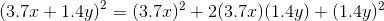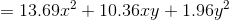## Example Questions

### Example Question #1 : Squaring / Square Roots / Radicals

Evaluate the following expression: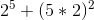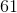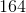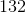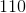Explanation:

2 raised to the power of 5 is the same as multiplying 2 by itself 5 times so:

25 = 2x2x2x2x2 = 32

Then, 5x2 must first be multiplied before taking the exponent, yielding 102 = 100.

100 + 32 = 132

### Example Question #2 : Square Of Sum

Expand: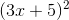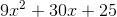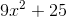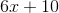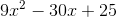Explanation:

To multiply a difference squared, square the first term and add two times the multiplication of the two terms. Then add the second term squared.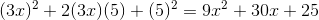### Example Question #3 : Square Of Sum

Which of the following is the square of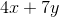?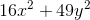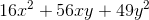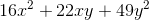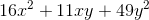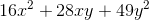Explanation:

Use the square of a sum pattern, substituting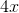forand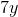forin the pattern: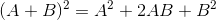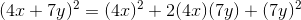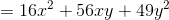### Example Question #1 : How To Find The Square Of A Sum

Which of the following is the square of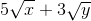?

You may assume bothandare positive.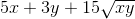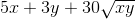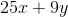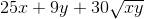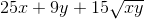Explanation:

Use the square of a sum pattern, substituting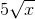forand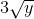forin the pattern: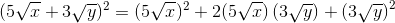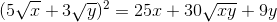or### Example Question #5 : Square Of Sum

Which of the following is the square of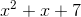?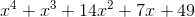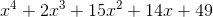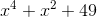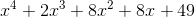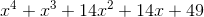Explanation:

Multiply vertically as follows: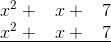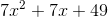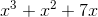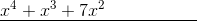### Example Question #6 : Square Of Sum

Which of the following is the square of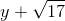?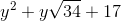The correct answer is not given among the other responses.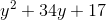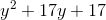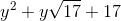The correct answer is not given among the other responses.

Explanation:

Use the square of a sum pattern, substitutingforand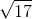forin the pattern: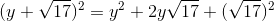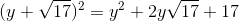This is not equivalent to any of the given choices.

### Example Question #7 : Square Of Sum

Which of the following is the square of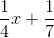?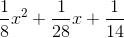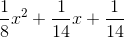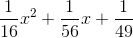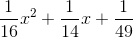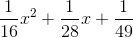Explanation:

Use the square of a sum pattern, substitutingforandforin the pattern: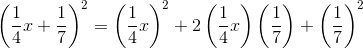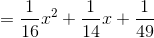### Example Question #8 : Square Of Sum

Which of the following is the square of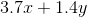?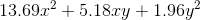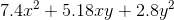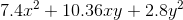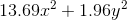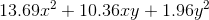Explanation:

Use the square of a sum pattern, substituting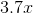forand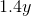forin the pattern: